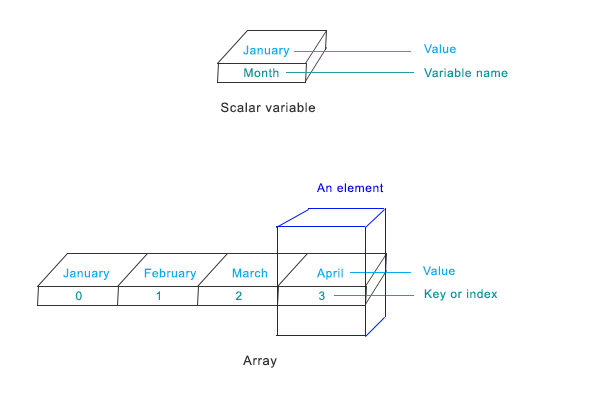# PHP Arrays## Why should you care array?

If you need to add a month name (ex. January) in your program, you’ll declare a variable name and assign the name in it. Like this-

```<?php
\$month=”January”;
?>```

Note, January is surrounded by quotes, it is a string type data.

Now, if you need to add all the month names, what you’ll do? You can do it in 2 ways-

Method 1: Declare 12 different variable names and assign month names in those variables. Like this-

```<?php
\$month=”January”;
\$month2=”February”;
\$month3=”March”;
\$month4=”April”;
\$month5=”May”;
\$month6=”June”;
\$month7=”July”;
\$month8=”August”;
\$month9=”September”;
\$month10=”October”;
\$month11=”November”;
\$month12=”December”;
?>```

But, it is a boring and time consuming task. There is a better solution, using method 2.
Method 2: Change the data type from string to array, add month names in it and assign it in a variable in the following way –

```<?php
\$month=array(“January”, “February”, “March” , “April” , “May” , “June” , “July” , “August” , “September” , “October” , “November” , “December”);
?>```

## What is an array?

An array is a special kind of data type that represents multiple values. In the above example, it represents 12 month names. Here, “month” is the array name. Please note that boolean, string, float, and integer each data type represents only one value; on the other hand, array represents multiply values.

## How variable stores array elements?

If you think variable as a container, then, the following picture shows how a scalar type variable (a scalar type variable contains boolean, integer, float, or string type data) and array type variable contain values.## Commonly used terms in array

• Element: Element of an array is the items it contains. An array can contain one or more elements.
• Value: Each element contains one value.
• Key or index: Key or index of an array is a unique number or a string that is associated with each value of an element.
• Length: The length of an array is the number of elements it contains.

## How to create an array:

There are 2 ways to create an array-

1. Using array() function.
Syntax
\$array_name = array(value1, value2, value3, …);
Example

`<?php \$month=array(“January”, “February”, “March” , “April” , “May” , “June” , “July” , “August” , “September” , “October” , “November” , “December”);?>`

Array created In the above way has automatic index numbers starts from 0 and increases by 1 for the subsequent values. So, the first value “January” has the index number 0, next one “February” has 1, and so on.

Manually created index number
There is a variation of the array() function when creating an array. In this way, index/key number can be mentioned when declaring the values.
Syntax
array_name = array(
key1 => value1,
key2 => value2,

)
Example

```<?php
\$month = array(
0 => “January”,
1 => “February”,
2 => “March”,
);?>```

Short array syntax
PHP 5.4 has introduced short array syntax. Now, array() can be written as [].If we write the previous example using [], it would be-
Syntax
array_name = [
key1 => value1,
key2 => value2,

);
Example

```<?php
\$month = &#91;
0 => “January”,
1 => “February”,
2 => “March”,
];?>```
2. Another way to create an array is using [] identifier.
Syntax
\$array_name[] = value;
Example

```<?php
\$month&#91;&#93;=“January”;
\$month&#91;&#93;=”February”;
\$month&#91;&#93;=”March”;
?>```

In the above example, January has index no. 0, February has 1 and March has 2.
Index/key can be assigned between []. Like this –
Syntax
\$array_name[key] = value;
Example

```<?php
\$month&#91;0&#93;=“January”;
\$month&#91;1&#93;=”February”;
\$month&#91;2&#93;=”March”;&#91;/php&#93;

?&gt;</li>
</ol>
<div class="note-nl">

<div>If you arbitrarily assign array keys. PHP won’t fill up the missing elements. In the following example, PHP will leave \$car and when you try to add a new element, PHP won’t insert that element in the \$car, instead, the key for the new element would be the next number of the existing highest key. Here, key for the new element “Rolse Royce” would be 4;
<?php
\$car&#91;0&#93;=’Ferray’;
\$car&#91;1&#93;=’Jaguar’;
\$car&#91;3&#93;=’Mercedes’;
\$car&#91;&#93;=’Rolls Royce’;
?>```

## How to change values of an array:

To change an existing value of an element, you need to specify the new value in the array mentioning the key of that element in [] brackets.
Syntax
\$array_name[existing_key] = “new_value”;
Example

```<?php
\$month = array(
0 => “January”,
1 => “February”,
2 => “March”,
)
array = “December”;
?>```

## How to delete an array:

To delete an array element or an entire array, unset() function can be used.

```<?php
\$car = array(“Ferarry”, “Huyandai”, “Toyota”, “Roles Royace”);
unset(car&#91;2&#93;); // Array element are now Ferarry, Huyandai, NULL, Roles Royace
unset(car); // Array element are now NULL
?>```

## Types of array

There are two types of array

1. Indexed array
2. Associative array

### Indexed array

Array with numeric keys/index is called indexed array. This is the most common type of array. The arrays that we used so far are all indexed array.

### Associative array

So far, you’ve seen number is used as key/index. Index can also be string too. This type of array is associative array.

```<?php
\$January = array(
“position” => “1st”,
“days” => 31,
“year” =>2013,
)
?>```

## Multidimensional array

When a key of an array represents another array it is called multidimensional array. In all the array examples above each key represents one value. In this sense, those are one-dimensional arrays.

```<?php
\$friends = array(
array(“John”, 20, “US”),
array(“Jack”, 25, “CA”),
)
?>```

## How to access an array element?

To print “January” from the array “month” above, you’ll write –
Syntax

`    echo \$array_name[key];`

Example

```<?php
echo \$month&#91;0&#93;;
?>```

Output: January
If key is not assigned, array key starts from 0, so, the first element stores January.

 ‹‹ PHP Strings : Previous Lesson Next Lesson: PHP Operators – Part1 ››